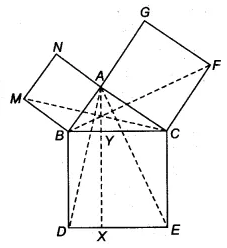Q

# In Fig. 9.34, ABC is a right triangle right angled at A. BCED, ACFG and ABMN are squares on the sides BC, CA and AB respectively. Line segment AX ⊥ DE meets BC at Y. Show that: (iv)

Q: 8     In Fig. , ABC is a right triangle right angled at A. BCED, ACFG and ABMN are squares on the sides BC, CA and AB respectively. Line segment   meets BC at Y. Show that:

(iv)Views[both 90]
By adding ABC  on both sides we get
ABC + FCA = ABC + BCE
FCB = ACE

In FCB and ACE
FC = AC [sides of square]
BC = AC [sides of square]
FCB = ACE

FCB    ACE

Hence proved

Exams
Articles
Questions